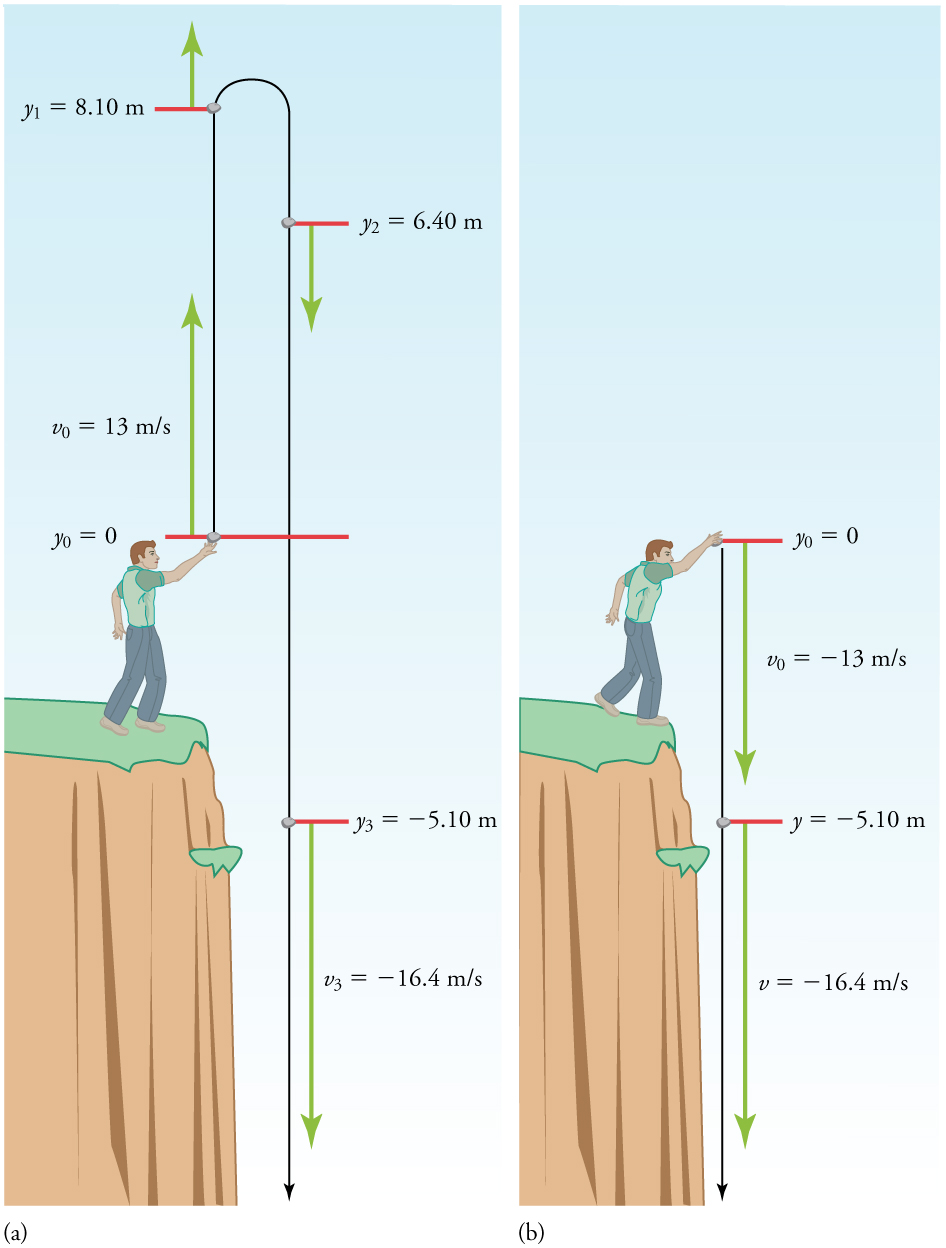# 2.7 Falling objects  (Page 4/9)

 Page 4 / 9

Solution

1. Identify the knowns. ${y}_{0}=0$ ; ${y}_{1}=-5\text{.}\text{10 m}$ ; ${v}_{0}=-\text{13}\text{.0 m/s}$ ; $a=-g=-9\text{.}\text{80 m}{\text{/s}}^{2}$ .

2. Choose the kinematic equation that makes it easiest to solve the problem. The equation ${v}^{2}={v}_{0}^{2}+2a\left(y-{y}_{0}\right)$ works well because the only unknown in it is $v$ . (We will plug ${y}_{1}$ in for $y$ .)

3. Enter the known values

${v}^{2}={\left(-\text{13}\text{.}\text{0 m/s}\right)}^{2}+2\left(-9\text{.}{\text{80 m/s}}^{2}\right)\left(-5\text{.}\text{10 m}-\text{0 m}\right)=\text{268}\text{.}{\text{96 m}}^{2}{\text{/s}}^{2},$

where we have retained extra significant figures because this is an intermediate result.

Taking the square root, and noting that a square root can be positive or negative, gives

$v=±\text{16}\text{.4 m/s}.$

The negative root is chosen to indicate that the rock is still heading down. Thus,

$v=-\text{16}\text{.4 m/s}.$

Discussion

Note that this is exactly the same velocity the rock had at this position when it was thrown straight upward with the same initial speed . (See [link] and [link] (a).) This is not a coincidental result. Because we only consider the acceleration due to gravity in this problem, the speed of a falling object depends only on its initial speed and its vertical position relative to the starting point. For example, if the velocity of the rock is calculated at a height of 8.10 m above the starting point (using the method from [link] ) when the initial velocity is 13.0 m/s straight up, a result of $±3\text{.}\text{20 m/s}$ is obtained. Here both signs are meaningful; the positive value occurs when the rock is at 8.10 m and heading up, and the negative value occurs when the rock is at 8.10 m and heading back down. It has the same speed but the opposite direction.(a) A person throws a rock straight up, as explored in [link] . The arrows are velocity vectors at 0, 1.00, 2.00, and 3.00 s. (b) A person throws a rock straight down from a cliff with the same initial speed as before, as in [link] . Note that at the same distance below the point of release, the rock has the same velocity in both cases.

Another way to look at it is this: In [link] , the rock is thrown up with an initial velocity of $\text{13}\text{.0 m/s}$ . It rises and then falls back down. When its position is $y=0$ on its way back down, its velocity is $-\text{13}\text{.0 m/s}$ . That is, it has the same speed on its way down as on its way up. We would then expect its velocity at a position of $y=-5\text{.}\text{10 m}$ to be the same whether we have thrown it upwards at $+\text{13}\text{.0 m/s}$ or thrown it downwards at $-\text{13}\text{.0 m/s}$ . The velocity of the rock on its way down from $y=0$ is the same whether we have thrown it up or down to start with, as long as the speed with which it was initially thrown is the same.

## Find g From data on a falling object

The acceleration due to gravity on Earth differs slightly from place to place, depending on topography (e.g., whether you are on a hill or in a valley) and subsurface geology (whether there is dense rock like iron ore as opposed to light rock like salt beneath you.) The precise acceleration due to gravity can be calculated from data taken in an introductory physics laboratory course. An object, usually a metal ball for which air resistance is negligible, is dropped and the time it takes to fall a known distance is measured. See, for example, [link] . Very precise results can be produced with this method if sufficient care is taken in measuring the distance fallen and the elapsed time.

Explain why a building made of bricks has smaller entropy than the same bricks in a disorganized pile. Do this by considering the number of ways that each could be formed (the number of microstates in each macrostate).
Omo physics hard ooo 😂😭😭😭
yeah but just need patient
Musbahu
The closeness of a measurement to the accepted value for a specific physical quantity is known as what ?
what's the procedure for solving exercise 9.
If you dive into water, you reach greater depths than if you do a belly flop. Explain this difference in depth using the concept of conservation of energy. Explain this difference in depth
A mass of 10kg move with a volocity of 4mß.find it's kinetic energy
80m/s²
Angel
KE=1/2mv²
Angel
m= 10
Angel
v=4
Angel
Happy to help
Angel
hi
Dike
What is the unit of force in F.P.S system
f=ma, f=m/s2
MD
lb-ft/s^2
Mary
what is an F.P.S system
Angel
please someone should send his or her number o. this platform
Pelumi
what is the unit for force
newtons
Angel
hello
Pelumi
Newton or kg. m/sec^2
Hiba
v=sin(att/s) Prove please as dimension consistent or not
v=sin(att/s) Prove please as dimension consistent or not
Hashim
what is physics
what is physic and what are its branches
= Kinematics = Quantum Physics = Dynamics = Statistics = Linear algebra = Calculus (Pre - Calculus, Calculus I Calculus II Calculus III Calculus IV) =Astrophysics And many more...... .....
puvananathan
what is andromeda
what is the milky way
What's the difference between deceleration and negative acceleration?
MNEMONICS FOR THE BRANCHES OF PHYSICS
are you asking for the mnemonics or you want to share it with us.?!?
paul
umar
While operating, a TV monitor is placed on its side during maintenance. The image on the monitor changes color and blurs slightly. Discuss the possible relation of these effects to the Earth’s magnetic field.
WhileGot questions? Get instant answers now! operating, a TV monitor is placed on its side during maintenance. The image on the monitor changes color and blurs slightly. Discuss the possible relation of these effects to the Earth’s magnetic field.
Dedachew

#### Get Jobilize Job Search Mobile App in your pocket Now!ByByBy Angela JanuaryBy Mistry BhaveshBy Marion CabalfinBy Brooke DelaneyBy Stephen VoronBy OpenStaxBy Brooke DelaneyBy Anh DaoBy OpenStaxBy OpenStax